Function Repository Resource:

# DivisorHasseDiagram

Generate a transitive reduction graph for the divisors of a positive integer

Contributed by: Shenghui Yang (Wolfram Research)
 ResourceFunction["DivisorHasseDiagram"][n] gives a Hasse diagram based on the divisors of n, represented as a Graph.

## Details and Options

A Hasse diagram is a directed graph representing a poset, with edges drawn based on the partial ordering. ResourceFunction["DivisorHasseDiagram"] uses the divisors as the graph's vertices and divisibility as the partial ordering.
ResourceFunction["DivisorHasseDiagram"] takes the same options as Graph.
ResourceFunction["DivisorHasseDiagram"] is efficient due to the slow growth of the Divisors function in length. So it does not suffer from the general NP-hardness of generating a directed TransitiveReductionGraph.

## Examples

### Basic Examples (2)

The Hasse diagram for the divisors of 12:

 In:=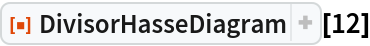Out=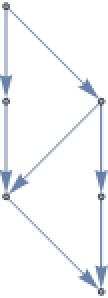In:=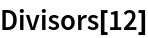Out=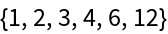The Hasse diagram for a prime number:

 In:=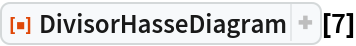Out=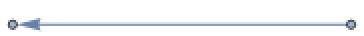### Properties and Relations (2)

For any prime power, DivisorHasseDiagram gives a linear graph where the number of vertices is one more than the exponent of the prime power:

 In:=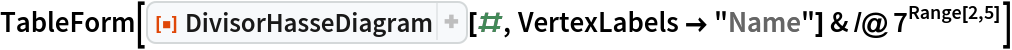Out=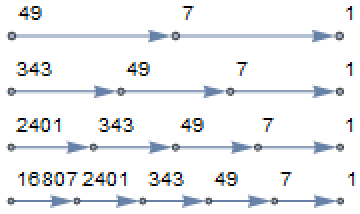The number of edges grows very slowly:

 In:=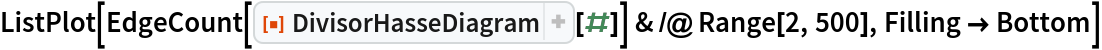Out=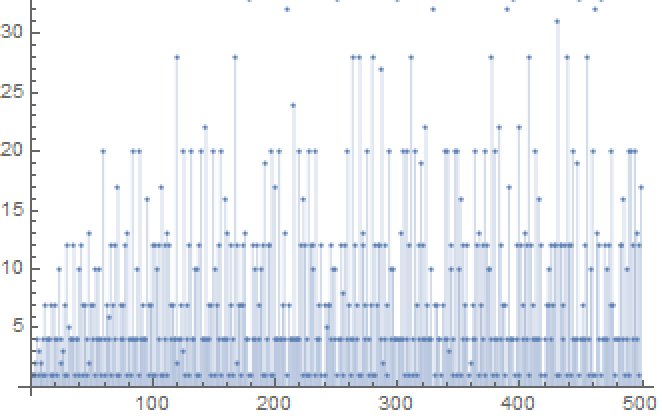### Neat Examples (3)

#### Hasse diagrams for some abundant numbers (3)

720 is an abundant number:

 In:=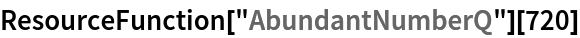Out=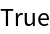Generate the Hasse diagram:

 In:=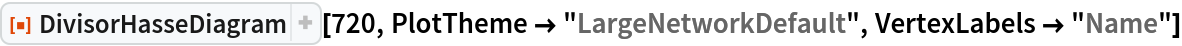Out=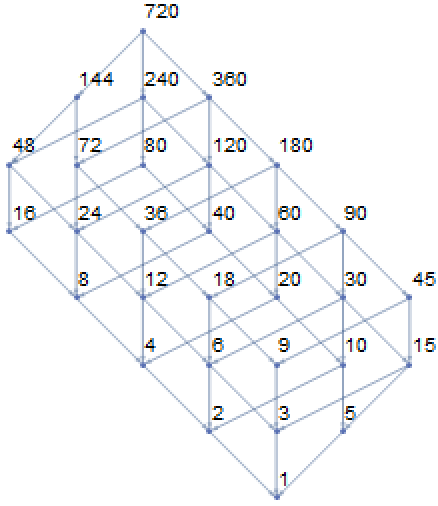2520 is an abundant number:

 In:=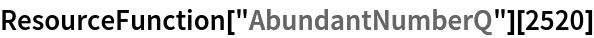Out=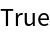Generate the Hasse diagram:

 In:=Out=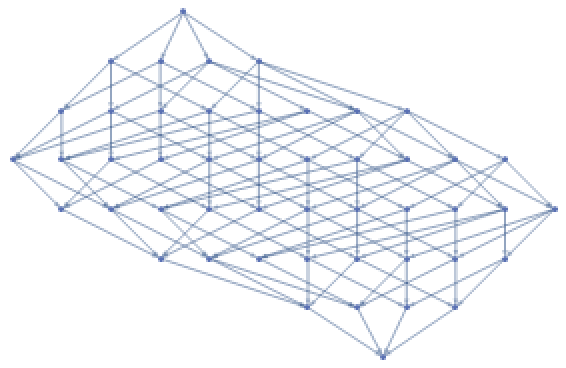21621600 is the only abundant number n that achieves equality in the inequality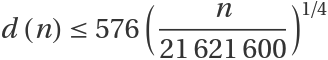, where d(n) is DivisorSigma[0,n] (the inequality was discovered by J.K. Nicolas):

 In:=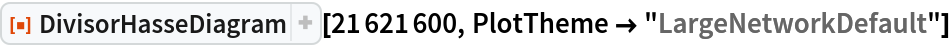Out=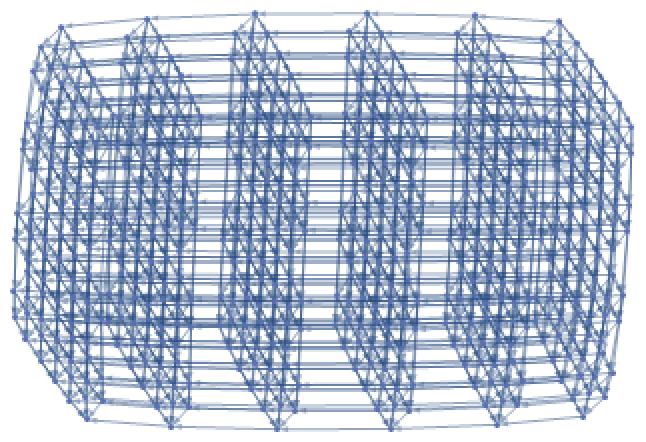Shenghui Yang

## Version History

• 1.0.0 – 27 May 2021